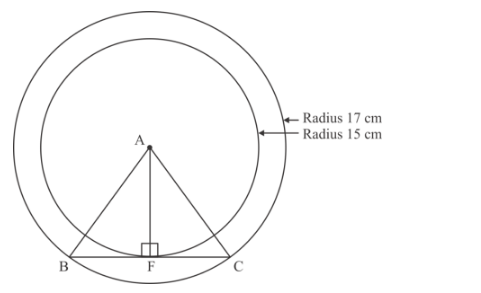# If radii of the two concentric circles are 15 cm and 17 cm,`
Question:

If radii of the two concentric circles are 15 cm and 17 cm, then the length of each chord of one circle which is tangent to other is:

(a) 8 cm

(b) 16 cm

(c) 30 cm

(d) 17 cm

Solution:

We are given that radii of two concentric circles are 15 cm and 17 cm

We have to find the length of each chord of one circle which is tangent to otherLet A be the centre of the two concentric circles

Let BC be the chord of bigger circle tangent to smaller at F

Therefore AF is the radius of smaller circleAB is the radius of bigger circleWe know that radius of a circle is perpendicular to its tangent

Therefore $A F \perp B C$

$\triangle A F B$

$\angle A F B=90^{\circ}$

Therefore

$B F=\sqrt{A B^{2}-A F^{2}}$ [Using Pythagoras theorem]

$=\sqrt{(17)^{2}-(15)^{2}} \mathrm{~cm}$

$=\sqrt{289-225} \mathrm{~cm}$

$=\sqrt{64} \mathrm{~cm}$

$=8 \mathrm{~cm}$

We know that a perpendicular from centre of circle to chord of circle bisects the chord

Therefore BF FC = 8 cm

Length of chord $=B F+F C$

$=8+8$

$=16 \mathrm{~cm}$

Hence option (b) is correct.